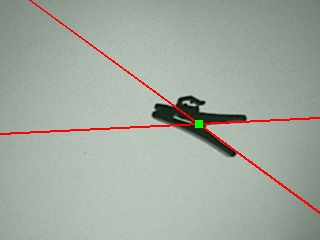# Calculate Intersection

The Calculate Intersection module provides a way to calculate the point at which two lines intersect. Note that if the lines are perfectly parallel the variables are removed as the lines will not intersect.

## Interface## Instructions

1. Line#1, Line #2 - Specify the variables that contain the start and end points (4 points define a line) for the two lines. Note that the notation variable_array:X can be used to access values within variable arrays.

2. Calculated Intersection - Specify the variables that will hold the resulting calculated intersection.

3. Shape, Color, Size - Specify the shape, color and size of the graphic that is used to indicate the point location.

4. Display as Annotation - Select if you want the graphic to be draw after all processing has been completed. If this is NOT selected then the next module in the processing pipeline will see the graphic as if it were part of the image and process it accordingly.

## Example

 Calculate_IntersectionClick Here to load a robofile that was used to produce the above intersection calculation using the Line Probe module to determine the lines.

 Calculate_Intersection Related Forum Posts Last post Posts Viewsnavigation by computer vision am writing a simple vbscript for navigation of car by computer vision ie. image processing i want to send serial c... 7 year 27 4658